# MCAT Physical : VSEPR Geometry

## Example Questions

### Example Question #1 : Vsepr Geometry

Which statement best describes VSEPR theory?

The repulsion between atoms helps determine the polarity of molecules

Covalent bonds are formed by overlapping valence electron shells

Molecular shapes are determined according to which orbitals are energetically accessible for bonding

The repulsion between protons in an atom's nucleus determines its size

The repulsion between electrons helps determine the geometry of covalent molecules

The repulsion between electrons helps determine the geometry of covalent molecules

Explanation:

The idea of VSEPR (valence shell electron pair repulsion) theory is that valence electron pairs repel each other, arranging themselves into positions that minimize their repulsions by maximizing the distance between them. The positions of these electron pairs then determine the overall geometry of the molecule. Molecular geometry is thus determined by the arrangement of electrons and nuclei such that the electrons are as far from one another as possible, while remaining as close to the positively charged nucleus as possible.

### Example Question #2 : Vsepr Geometry

Which of the following compounds has a molecular tetrahedral geometry?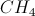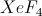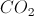Explanation:

Of the available answer choices, only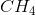has a tetrahedral geometry. Tetrahedral molecules have four constituents bound to the central without any lone pairs.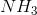has three bonds and a lone pair. It has a tetrahedral electronic geometry, but not molecular geometry.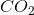has two double bonds, which give it a linear geometry.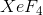has two lone pairs, giving the compound a square planar geometry.

### Example Question #3 : Vsepr Geometry

What is the molecular geometry of sulfur hexafluoride?

Tetrahedral

Square planar

Octahedral

Trigonal bipyramidal

Octahedral

Explanation:

Sulfur hexaflouride (SF6) is an example of octahedral geometry, as it follows the skeleton of AX6E0 format. A refers to sulfur, X to fluorine, and E to the lone pair electrons.

Square planar has an AX4E2 format, while tetrahedral and trigonal bipyramidal follow AXand AX5 formats, respectively.

### Example Question #4 : Vsepr Geometry

Which of the following is not the correct geometric configuration for the given molecule?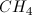, tetrahedral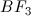, trigonal planar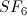, trigonal bipyramidal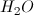, bent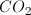, linear, trigonal bipyramidal

Explanation:

Recall the following relationships between geometry and number of pairs of electrons on the central atom.

2: linear

3: trigonal planar

4: tetrahedral

5: trigonal bipyriamidal

6: octahedral

To visualize the geometry, we need to think of how many electron pairs are on the central atom. Drawing Lewis dot diagrams may be helpful here. None of the answer choices has lone central electron pairs, with the exception of water, so the number of atoms bound to the central atom is the same as the number of central electron pairs.

The only one that does not match up with the correct geometry is SF6, which is actually octahedral since it has six central electron pairs. In a water molecule, the central oxygen has six valence electrons, plus one from each bond with hydrogen, for a total of eight central electrons and four central electron pairs. So, this geometry is a variation on the tetrahedral form (bent), in which two central electron pairs are not bound.

### Example Question #5 : Vsepr Geometry

Which of the following molecules will have the smallest bond angles?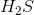Explanation:

In order to determine which molecule will have the smallest bond angle(s), make sure to factor in both the number of atoms around the central atom as well any lone pairs on the central atom.has two lone pairs around the central sulfur atom, which pushes the two hydrogens closer together than the ones found inand.

### Example Question #6 : Vsepr Geometry

The geometry of a certain molecule with the general formula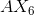is known to be octahedral. What is the hybridization?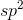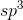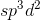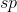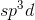Explanation:

Octahedral geometry always corresponds to thehybridization.hybridization corresponds to a general formula of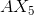hybridization corresponds to a general formula of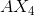hybridization corresponds to a general formula of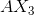, andhybridization corresponds to a general formula of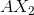.

### Example Question #7 : Vsepr Geometry

What is the geometry of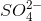?

Trigonal pyramidal

Square pyramid

Square planar

Tetrahedral

Seesaw

Tetrahedral

Explanation:

The central atom, sulfur, is surrounded by four electron groups (oxygen atoms), two of which are double bonded. Also note that the lone pairs are on the oxygen atoms, not the central atom. Thus the molecular geometry is tetrahedral.

### Example Question #8 : Vsepr Geometry

According to the VSEPR theory, what is the angle between the two lone pairs in?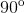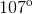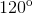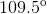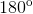According to VSEPR theory, the electron pairs will repel each other as much as possible. Therefore, in the octahedral shape, the lone pairs will be on opposite ends of the molecule, orfrom each other. For a molecule with a steric number of six (four atoms plus two lone pairs on the central atom), the basic geometry is octahedral. Since there are two lone pairs, the geometry becomes a slight variation of octahedral, square planar.